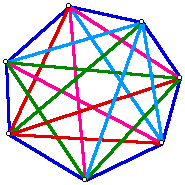# Diagonals in a Polygon

A diagonal is a line segment that connects non-adjacent vertices in a polygon. Consider the number of diagonals in a triangle, quadrilateral, pentagon, hexagon, heptagon, and octagon. What pattern do you notice? Use this pattern to predict the number of diagonals in a dodecagon (12-sided polygon).Hint
Consider comparing the number of vertices and the number of diagonals that can be drawn at each vertex.